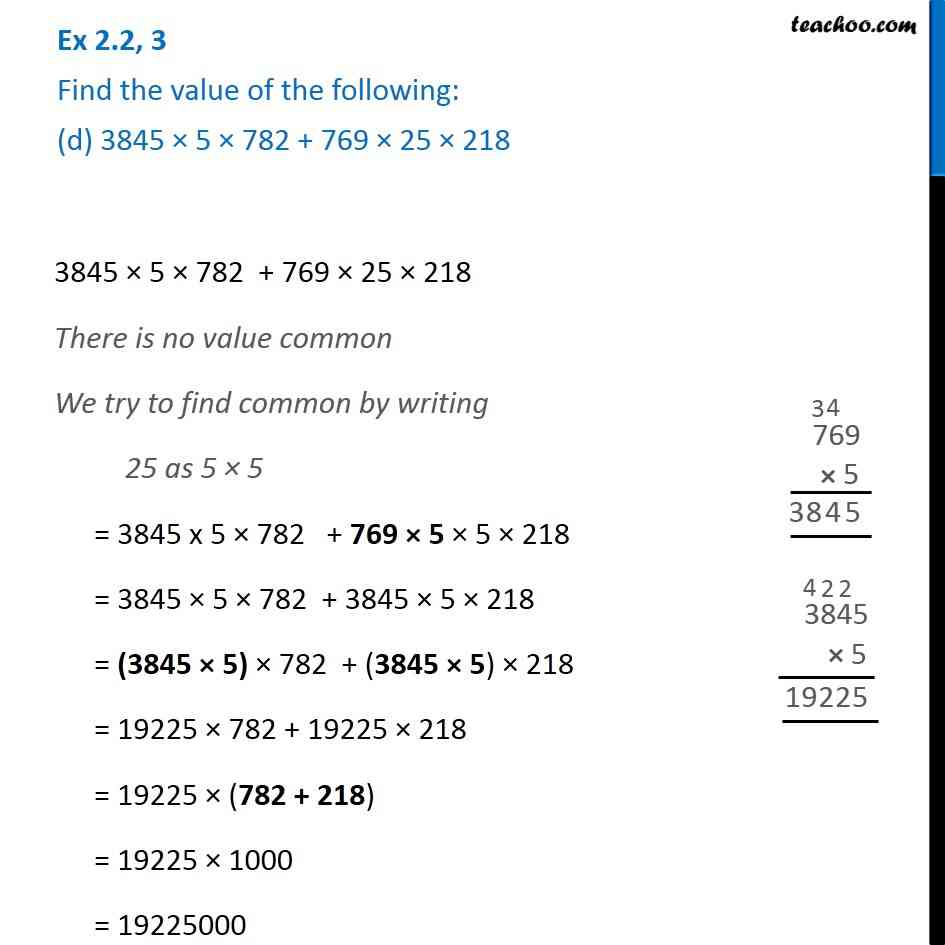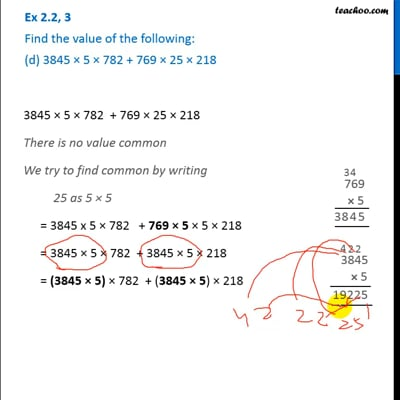Ex 2.2

Chapter 2 Class 6 Whole Numbers
Serial order wiseThis video is only available for Teachoo black users

Introducing your new favourite teacher - Teachoo Black, at only ₹83 per month

### Transcript

Ex 2.2, 3 Find the value of the following: (d) 3845 × 5 × 782 + 769 × 25 × 218 3845 × 5 × 782 + 769 × 25 × 218 There is no value common We try to find common by writing 25 as 5 × 5 = 3845 x 5 × 782 + 769 × 5 × 5 × 218 = 3845 × 5 × 782 + 3845 × 5 × 218 = (3845 × 5) × 782 + (3845 × 5) × 218 = 19225 × 782 + 19225 × 218 = 19225 × (782 + 218) = 19225 × 1000 = 19225000